# Sector in the theory of ordinary differential equations

(diff) ← Older revision | Latest revision (diff) | Newer revision → (diff)

An open curvilinear sectorwith vertex at an isolated singular point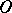of an autonomous system of second-order ordinary differential equations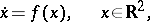(*)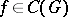, whereis the domain of uniqueness, that satisfies the following four conditions: 1) each lateral boundary ofis a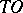-curve of the system (*) (i.e. a semi-trajectory that approachesas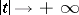, and touches a certain direction at); 2) the outer boundary ofis a simple parametric arc (the homeomorphic image of a closed interval); 3)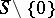does not contain singular points of (*). The fourth condition is one of the following three: 4a) all trajectories of the system (*) that start inleave this sector for both increasing and decreasing; such a sector is called a hyperbolic sector, or a saddle sector (Fig. a); 4b) all trajectories of (*) that start insufficiently neardo not leavebut approachasincreases, and asdecreases they leave(or vice-versa); such a sector is called a parabolic sector or an open node sector (Fig. b); or 4c) all the trajectories of (*) that start insufficiently neardo not leaveasincreases or decreases but approach, forming together withclosed curves (loops), and for any two loops one encloses the other; such a sector is called an elliptic sector or a closed node sector (Fig. c).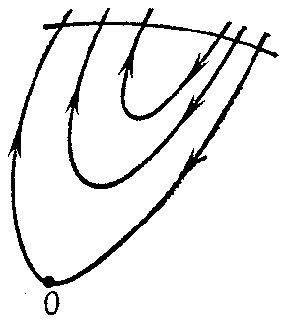Figure: s083770a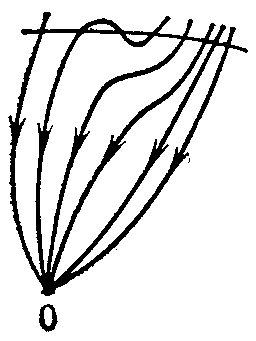Figure: s083770b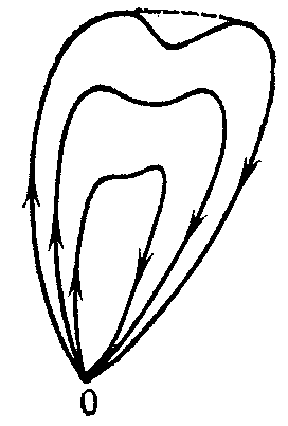Figure: s083770c

For any analytic system (*) with-curves, a discof sufficiently small radius and centre atcan always be divided into a finite number of sectors of a specific form:hyperbolic,parabolic andelliptic ones (see  and ). The Frommer method can be used to exhibit all these sectors, to determine the type of each, and to establish the rules of their succession in a circuit aboutalong the boundary of(and thereby to show the topological structure of the arrangement of the trajectories of (*) in a neighbourhood of). There are a priori estimates from above for,andin terms of the order of smallness of the normas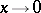(see , , ).

Sometimes (see, for example, ) the notion of a "sector" is defined more freely: In hyperbolic and parabolic sectors loops are allowed that cover a set without limit points on the rear boundary of a sector, and in elliptic sectors, loops that do not contain one another. Here the first sentence of the previous paragraph remains valid also for a system (*) of general form, and the Poincaré indexof the singular pointof (*) is expressed by Bendixson's formula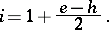How to Cite This Entry:
Sector in the theory of ordinary differential equations. Encyclopedia of Mathematics. URL: http://encyclopediaofmath.org/index.php?title=Sector_in_the_theory_of_ordinary_differential_equations&oldid=14776
This article was adapted from an original article by A.F. Andreev (originator), which appeared in Encyclopedia of Mathematics - ISBN 1402006098. See original article